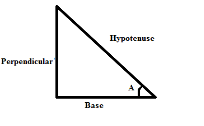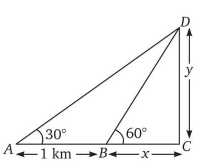# How to prepare Geometry and Trigonometry for RRB group D

RRB Group D exam conducted by the Railway Recruitment Board gives an opportunity for the aspirants to secure a lucrative career. In the blog we will be discussing the preparation strategy for Geometry and Trigonometry of Maths for Railways group D exam.

Attempt a FREE mock test of RRB Group D

## Maths for Railways group D exam (Topics: Geometry and Trigonometry)

During the preparation of RRB Mathematics, Geometry and Trigonometry are mostly neglected because of the fear associated with it. These topics are easy but with a right approach. Let’s first go through the subtopics from which the questions might be asked.

#### Geometry

• Basic problems on Polygons
• Basic problems on Circles
• Co-ordinate Geometry
• Mensuration

#### Trigonometry

• Identities
• Heights and Distances
• Measurement of Angles
• Maximum & Minimum values

After being accustomed with the subtopics the next step is to learn concepts, formulae and shortcuts for these topics. Maintaining short notes of these is important which will help in the last minute revision.

Accuracy and speed is a hurdle for many aspirants. Attempting and analysing sectional tests for geometry and trigonometry aids in increasing the speed and accuracy. The analysis of these tests also aid in learning new concepts.

Last but not the least; solve previous year questions on geometry and trigonometry to get acquainted with the question patterns.

## Practice Questions for Geometry and Trigonometry for RRB

1. If CotA = b/a, then find the value of Sec2A.

a) a2/b2

b) b2/a2

c) (1+ a2/b2)

d) (1+ b2/a2)

Solution:CotA = Base/Perpendicular = b/a

Hypotenuse = √(a2 + b2)

SecA = Hypotenuse/Base = √(a2 + b2)/b

Sec2A = [√(a2 + b2)/b]2 = (a2 + b2)/b2 = (1 + a2/b2)

Attempt a FREE mock test of RRB Group D

2. If the angles of elevation of a cloud from two consecutive kilometer-stones along a road are 30° and 60° respectively, the height of the cloud from the ground is:

a) 2/√3 km

b) √3/2 km

c) 3√3 km

d) 2√3 km

Solution:

Let the height of cloud from the ground be y km.In ΔBCD,

tan 60 = √3 = CD/BC = y/x

y = x√3 — (i)

In ΔACD,

tan 30 = 1/√3 = CD/AC = y/(x+1)

x + 1 = 3x [using eq (i)]

x = ½

Hence, height of cloud = √3/2 km

3. A man is walking around a rectangular park at a speed of 10km/hr. and completes one round in 40 minutes. What is the perimeter of the park?

a) 6.67km

b) 5.78 km

c) 3.37 km

d) 4.84 km

Solution:

The perimeter of the park = Distance covered in 40 minutes

= 10 * 40/60 = 6.67 km

Practice more such questions of Maths for Railways group D exam here.

4. A triangle has two of its angles in the ratio of 1:2. If the measure of one of its angles is 30 degrees, what is the measure of the largest angle of the triangle in degrees?

a) 900

b) 550

c) 1350

d) Cannot be determined

Solution:

The largest angle cannot be 30 degrees, if the smallest angle is 30 degrees, then the other angle would be 60 degrees and the largest angle would be 90 degrees. If the second largest angle is 30 degrees then the smallest angle would be 15 degrees and the largest angle would be 135 degrees. So, the largest angle cannot be determined. Hence, option d is correct.

5. Each exterior angle of a regular polygon is 45°. Find the number of sides of the polygon.

a) 8

b) 4

c) 2

d) 6

Solution:

Each exterior angle of a regular polygon = (360°/n), where n = no. of sides
So,

360°/n = 45°

n = 8

Practice more such questions of Maths for Railways group D exam here.

We hope this blog will aid you in your preparation for Geometry and Trigonometry in RRB Group D. All the best for the examination!